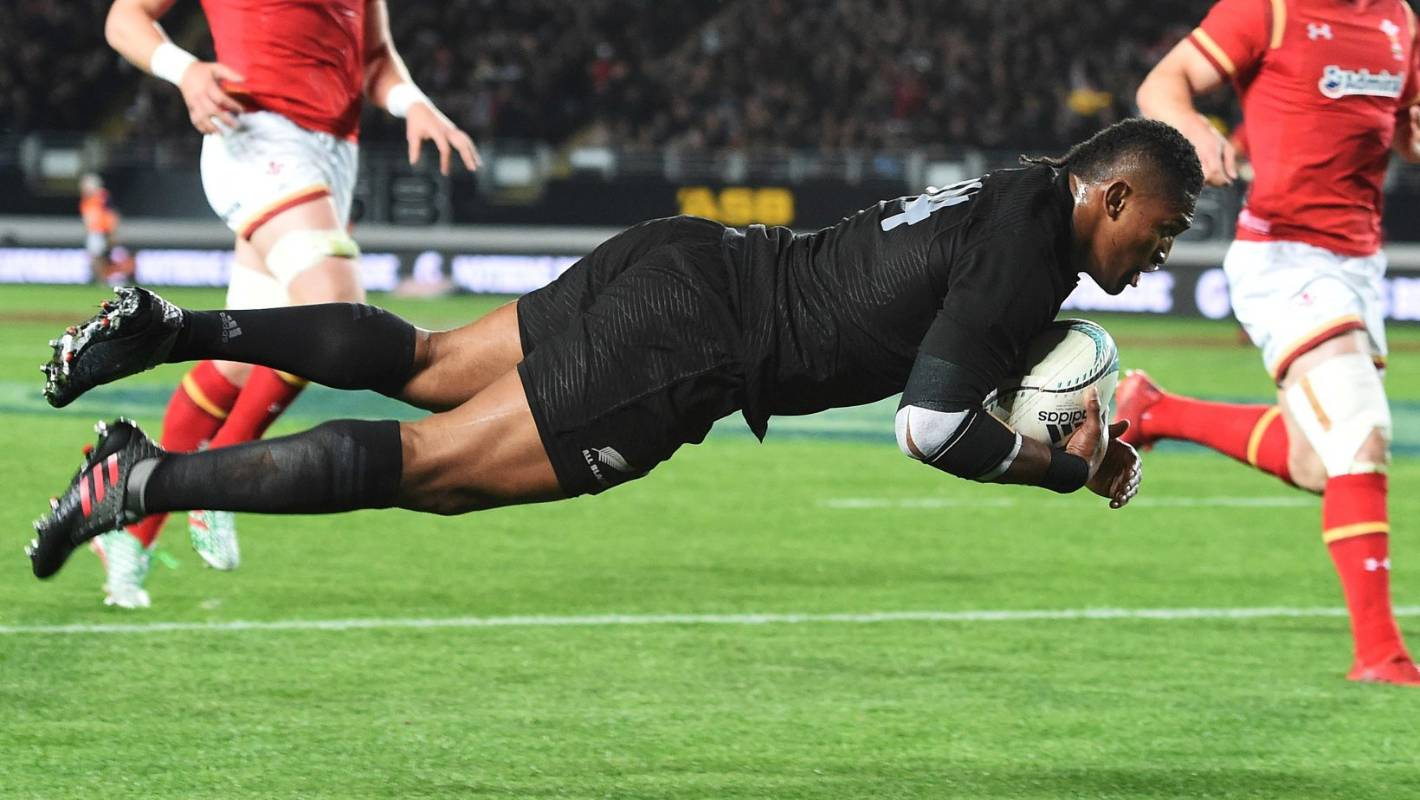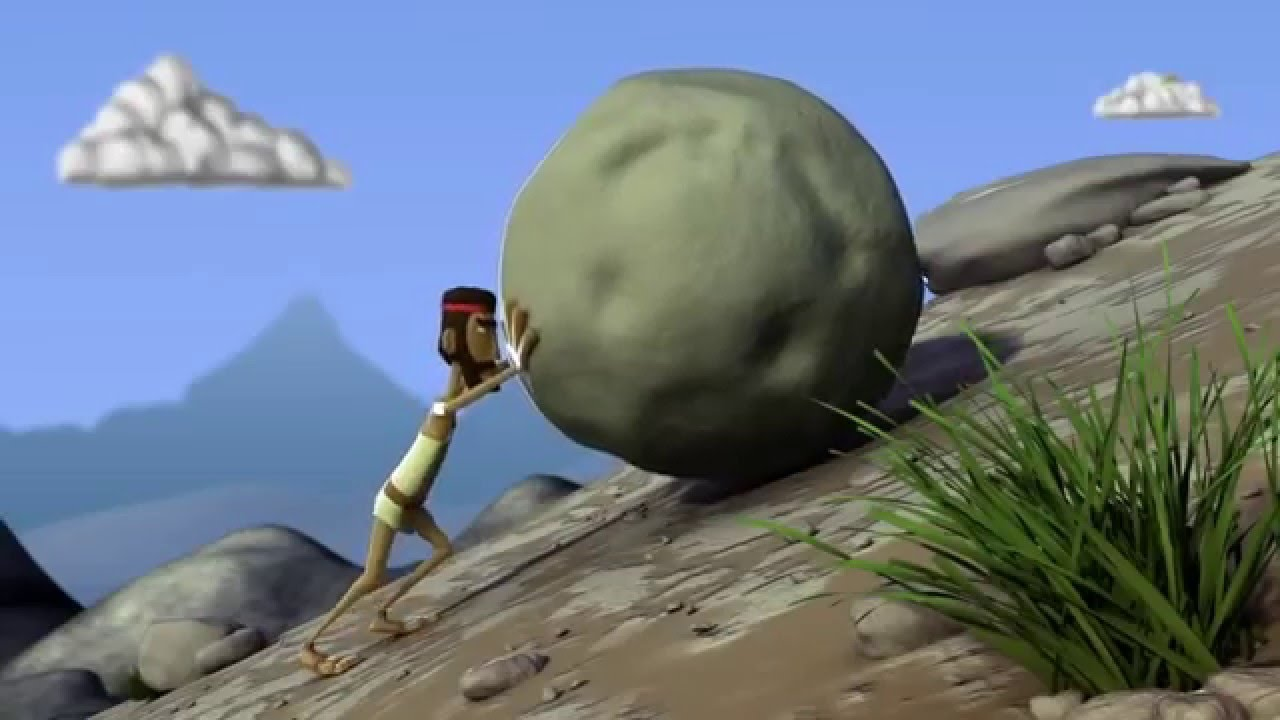Generally, force can bring

• Change in state of motion
• Change in shape

Let’s learn both

## Force and State of Motion

### What is state of motion?

It is the speed and direction of object.

If an object is moving ,it is in state of motion

If a person is not moving,it is at rest (in zero state of motion)

Hence, objects which are moving and which are not moving are both in state of motion

### How does Force bring change in state of motion

1. Force can make Moving Objects Stationary(It can stop moving objects)
2. Force can Move Stationary Objects (It can cause objects to move)
3. Force can change direction of object

#### Force can make Moving Objects Stationary

A goalkeeper drives to stop the ball

###(adsbygoogle = window.adsbygoogle || []).push({});

A fielder catches the ball#### Force can Move Stationary Objects (It can cause objects to move)

A footballer kicking a ballA person playing golf hits a ball#### Force can change direction of object

A batsman hitting a ball

Bowler bowls ball at the batsman

Batsman hits it in different directionA footballer passes ball to another footballerNote

#### Sometimes,there is no change in state of motion due to force

Example

If we push a heavy rock, it does not move

###This is because our force is of less magnitude  (size of our force is less)

#### Force can Change shape of Object

We can change shape of object by exerting force (putting force)

Example 1-Making chapati from dough

When we use dough for making chapatis, when we press the dough with our hands we apply force on the dough which changes its shape.Example 2-Squeezing Toothpaste

When we squeeze the toothpaste tube,its shape changes

This is because we apply force on the tube while squeezing it.## Questions

NCERT Question 2 - Give two examples of situations in which applied force causes a change in the shape of an object.

1. Class 8
2. Chapter 11 Class 8 - Force And Pressure
3. Concepts

Concepts

Class 8
Chapter 11 Class 8 - Force And Pressure